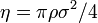# Liu hard disk equation of state

(diff) ← Older revision | Latest revision (diff) | Newer revision → (diff)
$1 + \eta^2/8 + \eta^4/18- \4eta^4/21}{(1-\eta)^2$
where the packing fraction is given by$\eta = \pi \rho \sigma^2 /4$ where$\sigma$ is the diameter of the disks.